# Linear Regression in Machine Learning

## Linear Regression in Machine Learning

In this article, I am going to discuss Linear Regression in Machine Learning with Examples. Please read our previous article where we discussed Machine Learning Fundamentals with Examples.

##### Linear Regression in Machine Learning

One of the most well-known and well-understood algorithms in statistics and machine learning is linear regression. Before we go into the specifics of linear regression, you might be wondering why we’re looking at it.

##### Isn’t it a statistical technique?

Machine learning, more especially predictive modeling, is primarily concerned with minimizing a model’s error or creating the most accurate predictions feasible at the sacrifice of explainability. In applied machine learning, we will borrow, reuse, and steal algorithms from a variety of domains, including statistics, and apply them to these purposes.

Linear regression is a type of linear model, which assumes a linear connection between the input variables (x) and a single output variable (y). That is, y can be determined using a linear combination of the input variables (x).

The procedure is known as simple linear regression when there is only one input variable (x). When there are many input variables, statistics literature frequently refers to the approach as multiple linear regression. Because of its simplicity, linear regression is an appealing model.

The representation is a linear equation that combines a specific set of input values (x) and yields the projected output for that set of input values (y). As a result, both the input (x) and output values are numeric.

The linear equation assigns one scale factor to each input value or column, denoted by the capital Greek letter Beta (B). One more coefficient is added, giving the line more freedom (e.g., moving up and down on a two-dimensional plot), and is often referred to as the intercept or bias coefficient.

For example, in a simple regression problem (a single x and a single y), the form of the model would be:

y = B0 + B1*x

In larger dimensions When there are multiple inputs (x), the line is referred to as a plane or a hyper-plane. The form of the equation and the precise numbers utilized for the coefficients are hence the representation (e.g. B0 and B1 in the above example)

It is usual to discuss the complexity of a regression model, such as linear regression. This relates to how many coefficients are utilized in the model.

When a coefficient reaches zero, it effectively removes the influence of the input variable on the model and, as a result, the model’s prediction (0 * x = 0). This is important when considering regularization strategies, which alter the learning algorithm to minimize the complexity of regression models by exerting pressure on the absolute magnitude of the coefficients, driving some to zero.

##### Simple Linear Regression in Machine Learning

Simple linear regression is a parametric test, which means it makes specific data assumptions.

1. Homogeneity of variance (homoscedasticity): the extent of the error in our prediction does not vary considerably among independent variable values.
2. Observation independence: the observations in the dataset were acquired using statistically acceptable sampling methods, and there are no hidden links between them.
3. Normality: The data is distributed normally.

One more assumption is made by linear regression:

1. The independent and dependent variables have a linear relationship: the best fit line through the data points is a straight line (rather than a curve or some sort of grouping factor).

The formula for a simple linear regression is: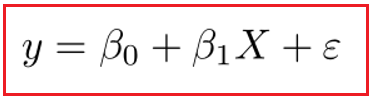• y is the predicted value of the dependent variable (y) for any given value of the independent variable (x).
• B0 is the intercept, the predicted value of y when the x is 0.
• B1 is the regression coefficient – how much we expect y to change as x increases.
• x is the independent variable (the variable we expect is influencing y).
• e is the error of the estimate, or how much variation there is in our estimate of the regression coefficient.

Linear regression determines the best fit line across your data by looking for the regression coefficient (B1) that minimizes the model’s total error (e).

##### Multiple Linear Regression in Machine Learning

To assess the association between two or more independent variables and one dependent variable, multiple linear regression is utilized. You can use multiple linear regression to find out:

1. The degree to which two or more independent variables are related to one dependent variable (e.g. how rainfall, temperature, and amount of fertilizer added affect crop growth).
2. The dependent variable’s value at a given value of the independent variables (e.g. the expected yield of a crop at certain levels of rainfall, temperature, and fertilizer addition).

The formula for multiple linear regression is: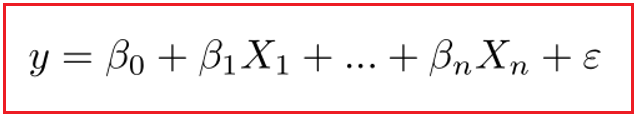• y = the predicted value of the dependent variable
• B0 = the y-intercept (value of y when all other parameters are set to 0)
• B1X1= the regression coefficient (B1) of the first independent variable (X1) (a.k.a. the effect that increasing the value of the independent variable has on the predicted y value)
• … = do the same for however many independent variables you are testing
• BnXn = the regression coefficient of the last independent variable
• e = model error (a.k.a. how much variation there is in our estimate of y)

Multiple linear regression computes three things to obtain the best-fit line for each independent variable:

• The regression coefficients with the lowest total model error.
• The entire model’s t-statistic.
• The corresponding p-value (how likely it is that the t-statistic would have occurred by chance if the null hypothesis of no relationship between the independent and dependent variables was true).
• The t-statistic and p-value are then computed for each regression coefficient in the model.

### Case Study in Finance Domain

##### Introduction

According to FCI’s Global Industry Activity Report 2019, the share of international factoring by country was roughly 22 percent, with domestic factoring accounting for the remaining 78 percent – hence, as previously said, a typical country would exhibit these proportions. These proportions have remained practically constant over the previous three years.

By far the largest contribution to FCI Members’ Domestic Volume comes from Europe. Non-Recourse volume has increased to 40%, Recourse volume has increased to 23%, and Invoice Discounting has decreased to 22%.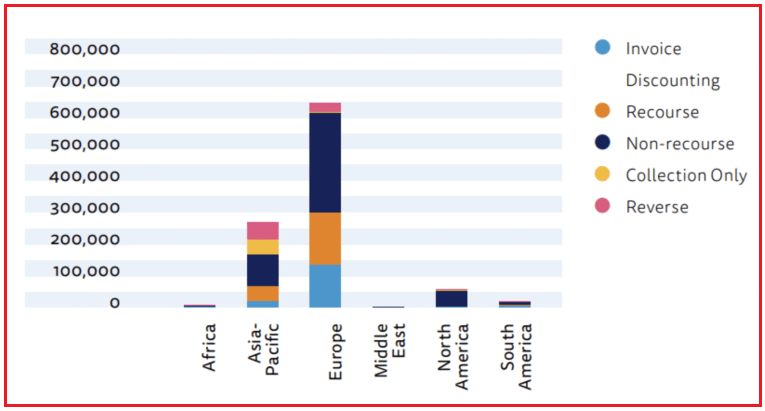When the volumes of FCI Members International are broken down by continent, Europe, with 61 percent, has surpassed Asia Pacific (34 percent). The Americans accounted for more than 5%, whereas Africa and the Middle East accounted for less than 1%.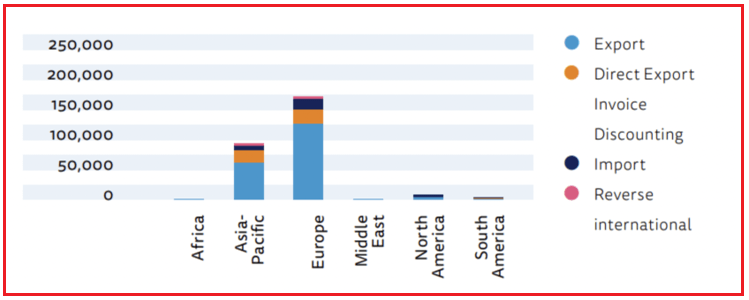##### Problem Statement

The most difficult aspect of factoring is predicting whether or not an invoice will be paid. By purchasing the invoice, the factor gives money against the future payment to the business owners. The factor then receives the cash and applies its interest rate or discounts to it (if acceptable). The factor loses its advance cash if the invoice is not paid.

```Importing Libraries
# For Panel Data Analysis
import pandas as pd
from pandas_profiling import ProfileReport
pd.set_option('display.max_columns', None)
pd.set_option('display.max_rows', None)
pd.set_option('mode.chained_assignment', None)

# For Numerical Python
import numpy as np

# For Scientific Python
from scipy import stats

# For random numbers
from random import randint

# For Data Visualization
import matplotlib.pyplot as plt
%matplotlib inline
import seaborn as sns

# For Preprocessing
from sklearn.preprocessing import LabelEncoder, OneHotEncoder, StandardScaler

# For Feature Selection
from sklearn.feature_selection import SelectFromModel

# For metrics evaluation
from sklearn.metrics import mean_squared_error, r2_score

# For Data Modeling and Evaluation
from sklearn.model_selection import train_test_split
from sklearn.linear_model import LinearRegression, Ridge, Lasso
from sklearn.ensemble import RandomForestRegressor

# To Disable Warnings
import warnings
warnings.filterwarnings(action = "ignore")
print('Data Shape:', data.shape)
```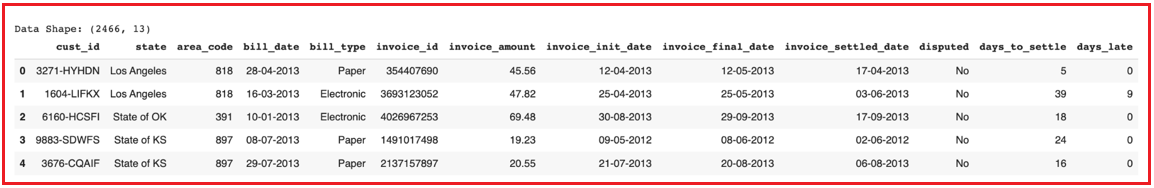##### Data Description

In this section we will get information about the data and see some observations.

data.describe()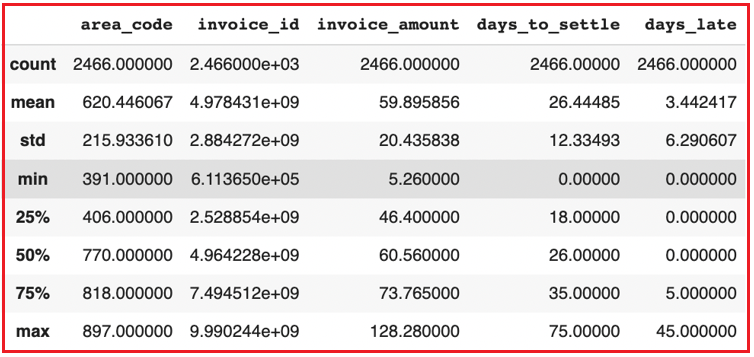data.info()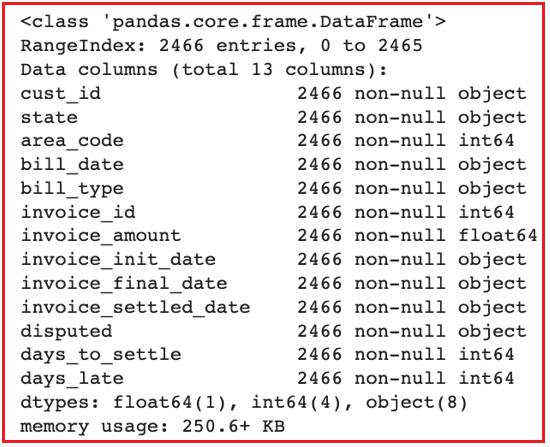##### Data Pre-Processing
```data['bill_date'] = pd.to_datetime(data['bill_date'])
data['invoice_init_date'] = pd.to_datetime(data['invoice_init_date'])
data['invoice_final_date'] = pd.to_datetime(data['invoice_final_date'])
data['invoice_settled_date'] =pd.to_datetime(data['invoice_settled_date'])
```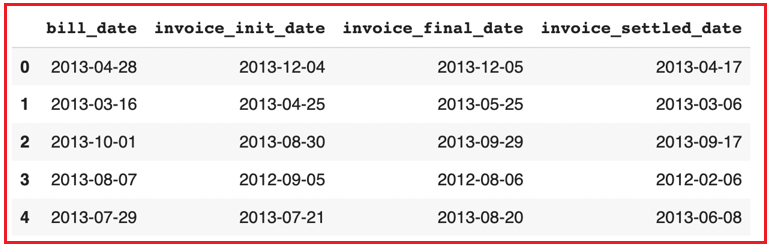##### EDA

We will create some new features out of the existing ones which can help out in our analysis. These will be –

1. late: It will show whether a customer was late or not.
2. count_late:It will show the number of times a certain customer was late.
3. invoice_quater It signifies quarter of the date in invoice_init_date.
4. repeat_cust It will show the frequency of repeated customers.
5. binned_invoice_amount: This feature is derived from invoice amount signifying categories of invoice amount i.e. Less than 60 or Greater than 60.
```data['late'] = data['days_late'].apply(lambda x: 1 if x>0 else 0)
data['count_late'] = data['late'].eq(1).groupby(data['cust_id']).apply(lambda x: x.cumsum().shift().fillna(0)).astype(int)
data['invoice_quater'] = data['invoice_init_date'].dt.quarter

# For frequency of repeated customer
temp_data = data[data['late'] == 1].groupby(['cust_id'], as_index = False)['days_late'].count()
temp_data.columns = ['cust_id', 'repeat_cust']
data = pd.merge(data, temp_data, how = 'left', on = 'cust_id')
data['repeat_cust'].fillna(0, inplace=True)
data['repeat_cust'] = data['repeat_cust'].astype('int')
data['binned_invoice_amount'] = data['invoice_amount'].apply(lambda x: 'More than 60' if x > 69 else 'Less than 60')
print('Data Shape:', data.shape)
```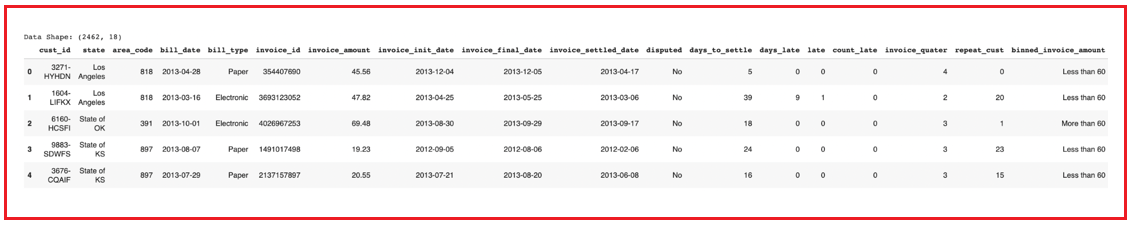##### Question 1: What is the average frequency of getting late with respect to area code?
```data2 = pd.DataFrame(data.groupby(['area_code'], as_index = False)['days_late'].mean())
plt.figure(figsize = [10, 6])
sns.barplot(x = 'area_code', y = 'days_late', data = data2, linewidth = 2.5)
plt.xlabel('Area Code', size = 14)
plt.ylabel('Days Late', size = 14)
plt.title('Average Days Late vs Area Code', size = 16)
plt.show()
```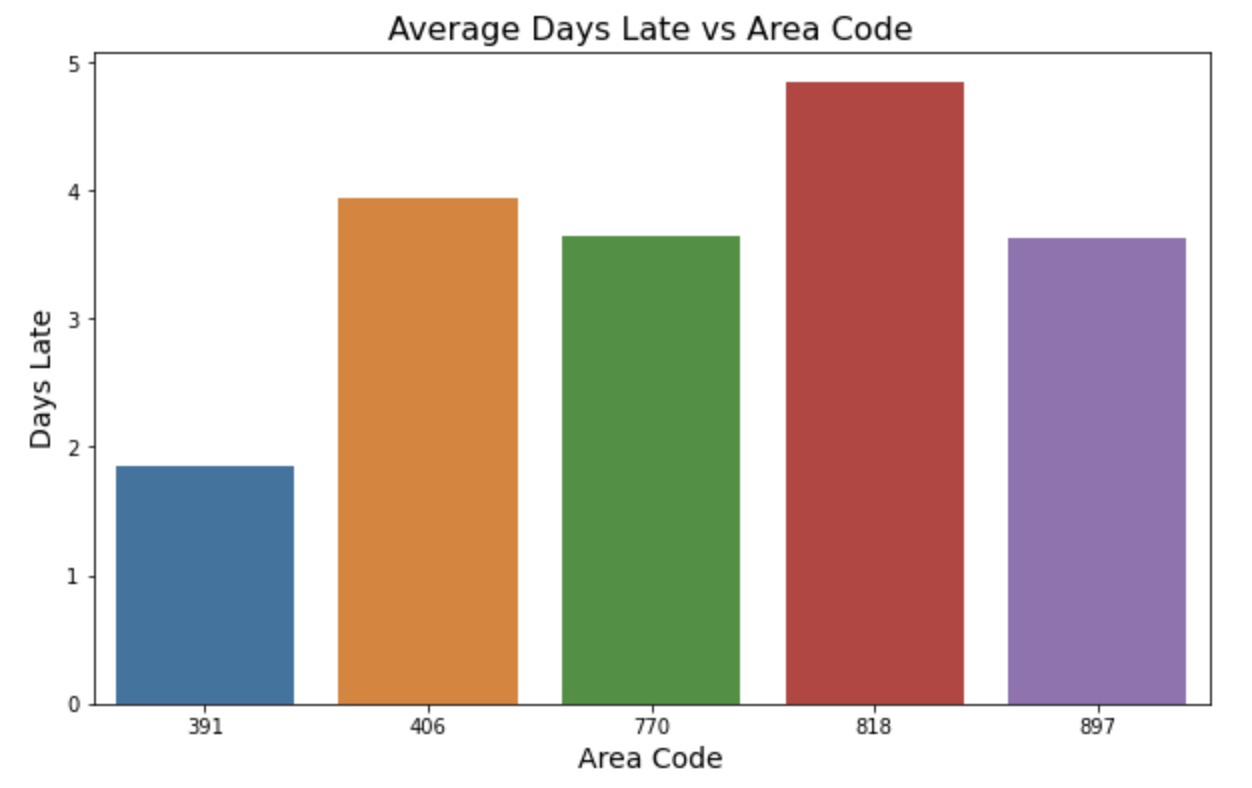##### Question 2: What is the proportion of transactions with respect to being late or not?
```print('Late Transactions:', len(data[data['late'] == 1]))
print('On Time Transactions:', len(data[data['late'] == 0]))

data['late'].value_counts().plot(kind = 'pie', explode = [0.1, 0], fontsize = 14, autopct = '%3.1f%%',
wedgeprops = dict(width=0.15), shadow = True, startangle = 160,
figsize = [13.66, 7.68], legend = True)
plt.legend(['On Time', 'Late'])
plt.ylabel('Category')
plt.title('Proportion of late transactions', size = 14)
plt.show()
```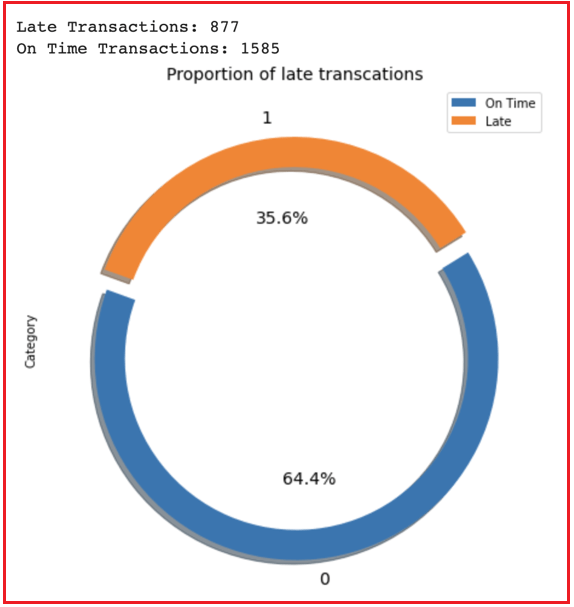##### Question 3: What is the average frequency of repeated transactions with respect to days late?
```data_temp = pd.DataFrame(data.groupby(['repeat_cust'], as_index = False)['days_late'].mean())
plt.figure(figsize = [12, 5])
sns.barplot(x = 'repeat_cust', y = 'days_late', data = data_temp, palette = sns.color_palette("Blues", n_colors= 32))
plt.xlabel('Repeated Customer', size = 14)
plt.ylabel('Days Late', size = 14)
plt.title('Frequency Distribution of Repeated Customers (Average)', size = 16)
plt.show()
```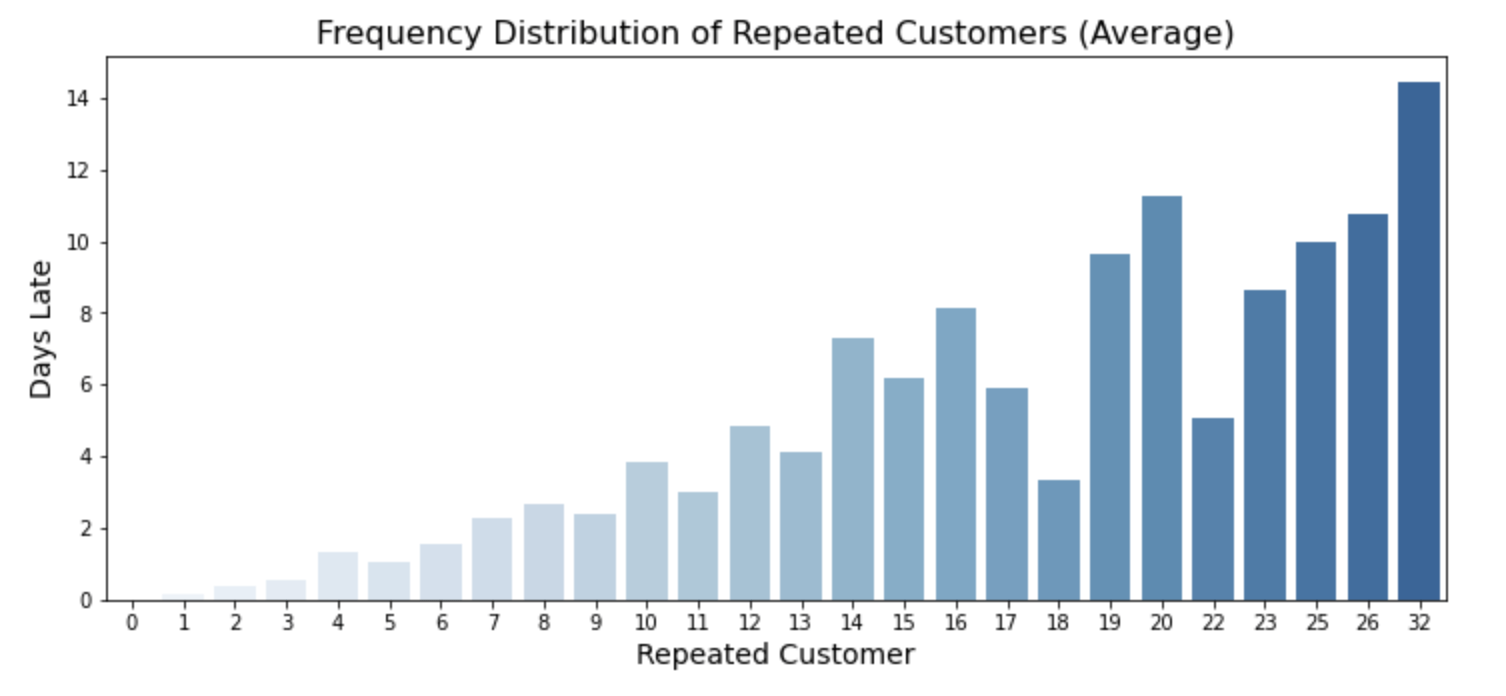##### Feature Correlation
```figure = plt.figure(figsize = [12, 6])
sns.heatmap(data.corr(), annot = True, cmap = 'viridis')
plt.title('Correlation between Features', size = 14)
plt.show()
```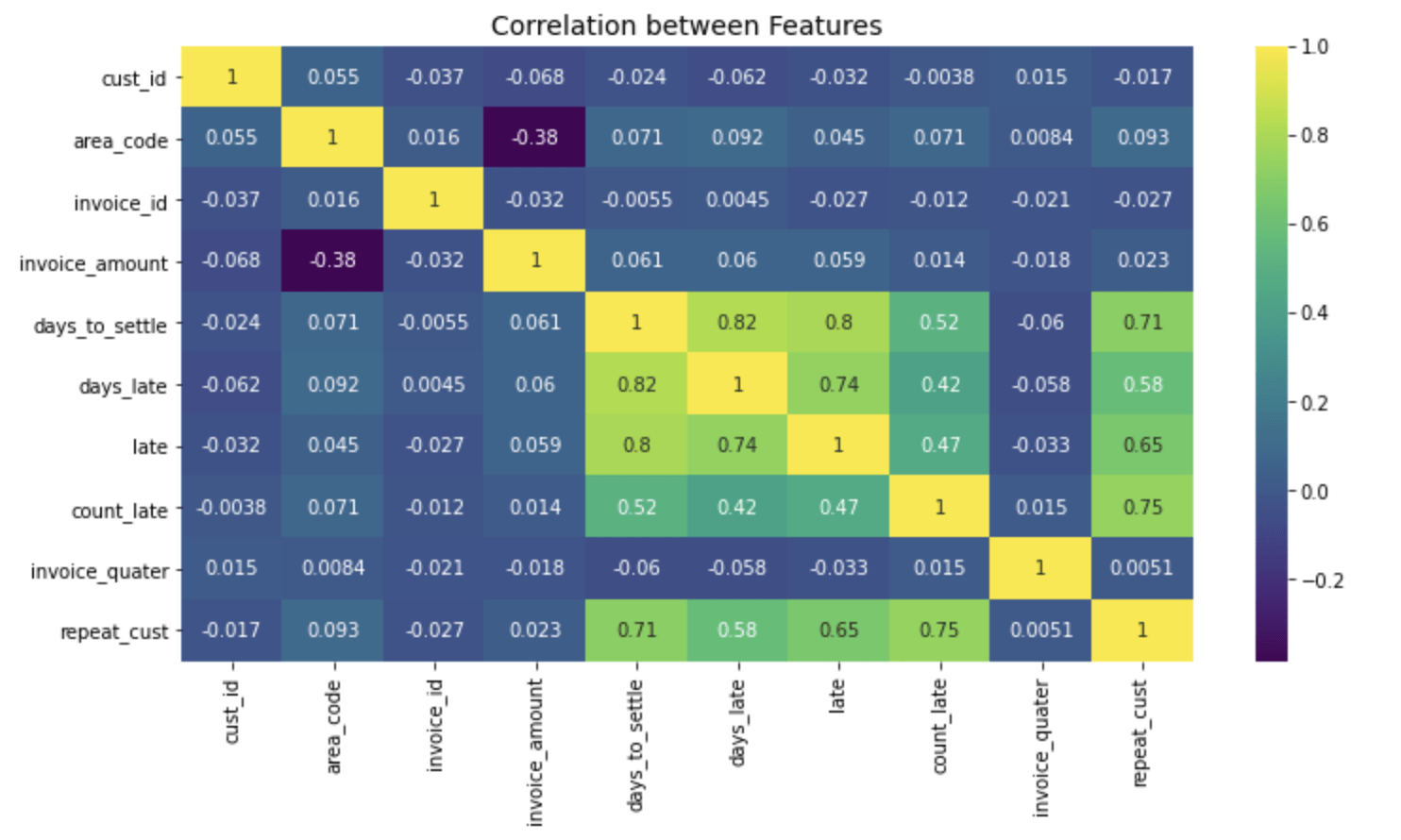##### Label Encoding
```ordered_labels = ['bill_type', 'disputed', 'binned_invoice_amount']
encode = LabelEncoder()

for i in ordered_labels:
if isinstance(data[i].dtype, object):
data_encoded[i] = encode.fit_transform(data_encoded[i])
print('Label Encoding Success!')
print('Data Shape:', data_encoded.shape)
```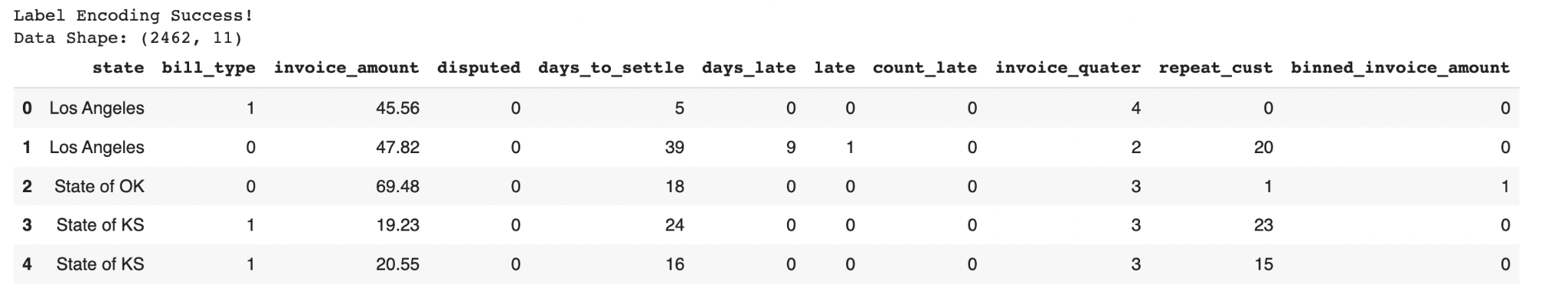```data_encoded = pd.get_dummies(data = data_encoded, columns = ['state'])
print('Data Shape:', data_encoded.shape)
```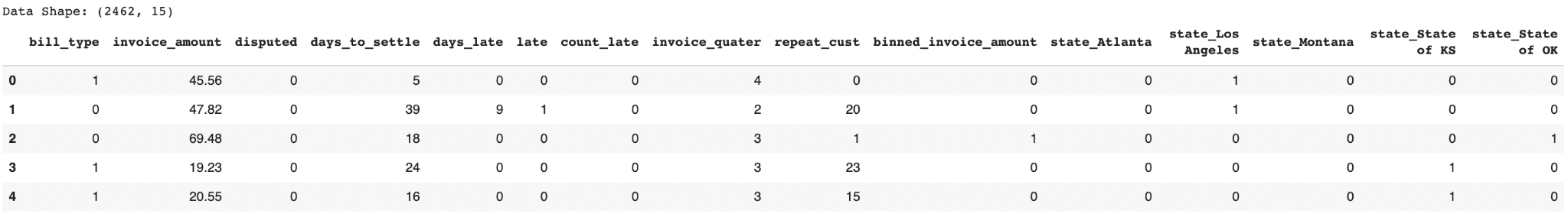X_train, X_test, y_train, y_test = train_test_split(X, y, test_size = 0.2, random_state = 42)

##### Model Building
```lr = LinearRegression()
lr.fit(X_train, y_train)
y_train_pred = np.abs(lr.predict(X_train).astype('int'))
y_test_pred = np.abs(lr.predict(X_test).astype('int'))

print('Actual Values:', y_test[0:5])
print('Predicted Values:', y_test_pred[0:5])
# Estimating RMSE on Train & Test Data
print('RMSE (Train Data):', np.round(np.sqrt(mean_squared_error(y_true = y_test, y_pred = y_test_pred)), decimals = 2))
print('RMSE (Test Data):', np.round(np.sqrt(mean_squared_error(y_true = y_test, y_pred = y_test_pred)), decimals = 2))
# Estimating R-Squared on Train & Test Data
print('R-Squared (Train Data):', np.round(lr.score(X_train, y_train), decimals = 2)*100, '%')
print('R-Squared (Test Data):', np.round(lr.score(X_test, y_test), decimals = 2)*100, '%')
# Plotting Actual vs Predicted values
PlotScore(y_train, y_train_pred, y_test, y_test_pred)
```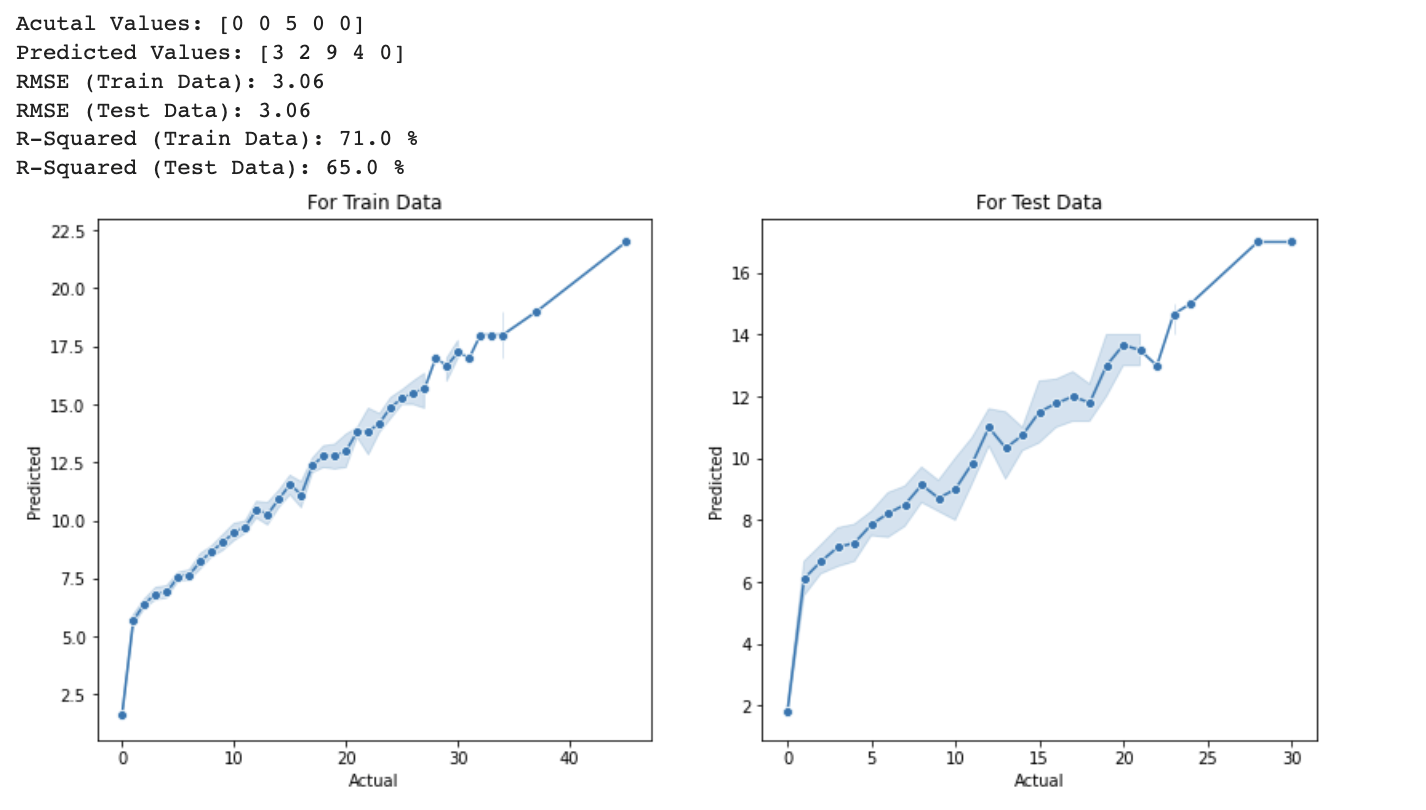In the next article, I am going to discuss Logistic Regression in Machine Learning with Examples. Here, in this article, I try to explain Linear Regression in Machine Learning with Examples. I hope you enjoy this Linear Regression in Machine Learning with Examples article.